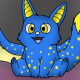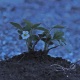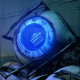# ComputerCraft | Programmable Computers for Minecraft

»

##ds8418214 May 2013manawyrm, on 10 May 2013 - 12:32 PM, said:

Hello,

have you still got a binary for the 1.4.7 version?
I tried to build it from the source, but failed at compiling.

Thanks,
Tobias
No. I do not have a 1.4.7 binary. The current version cannot be ported either, because it uses new forge hooks. Sorry.

##manawyrm14 May 2013

hmm. It can be done...
It worked fine, I only had a weird bug on ForgeBukkit that caused my client's to crash, if I was setting the pixels to fast...

##allquan14 May 2013

Yeah love the new CC lights 2! I hope you will continue this gigant work##Tiin5701 Jun 2013

By the way...
CCLights2 for 1.5.2:
[REDACTED -tiin57]
Totally untested, I just recompiled it in MCP 7.51.
Please don't bother reporting bugs for this version, since I'm not offering support for it and ds84182 didn't have anything to do with it.

##ArchAngel07508 Jun 2013

this is absolutely brilliant!, if one can make a game with models that define their own shape and colors then a highly detailed graphical game can be made!
i might just abuse this!

Love the program, and love how making a 128*128 screen randomise each and every pixel every 0.1 doesnt kill my pc XD

that's meant for cclights1

Going to start abusing CClights2 and see what crazy i can get up to!

##MindenCucc04 Jul 2013ds84182, on 05 February 2013 - 12:31 PM, said:

This is for 1.4.7 MC? Because I tried almost every forge for 1.4.7, and it just keeps crashing with this:
```java.lang.VerifyError:
(class: ds/mods/CCLights2/PacketHandler, method: onPacket
Data signature: (Lcg;Ldk;Lcpw/mods/fml/common/network/Player;)V)
Incompatible object argument for function call
```

##ds8418211 Jul 2013MindenCucc, on 04 July 2013 - 10:21 AM, said:ds84182, on 05 February 2013 - 12:31 PM, said:

This is for 1.4.7 MC? Because I tried almost every forge for 1.4.7, and it just keeps crashing with this:
```java.lang.VerifyError:
(class: ds/mods/CCLights2/PacketHandler, method: onPacket
Data signature: (Lcg;Ldk;Lcpw/mods/fml/common/network/Player;)V)
Incompatible object argument for function call
```
I forgot to update the post after the recent forum rollback.
It is for 1.5.2.

##Wiiplay12311 Aug 2013

Where is the CCDesk version of it?

##Shaun23 Aug 2013

What's the current status of this mod? Are there recipes for survival mode? Are crashes/other bugs fixed? What's the bandwidth load caused by the screens?

also, is there any way for these screens to accept keyboard/mouse input? can you hook multiple screens into one like with normal CC monitors?

sorry for all the questions but the OP doesn't give a lot of information.

Never mind.

##Pecacheu02 Nov 2013

I don't know how to use this mod at all.
Spoiler
I looked everywhere for a tutorial on CCLights or CCLights 2, but couldn't even find a spotlight!
I really need some help. I would also like to know how to use the "tablet"...

##Knux1408 Nov 2013

For notch's sake, please .setHardness() and .setResistance()

##karelmikie316 Nov 2013

can you include this mod in modpacks couldn't find anything about that?

##Maltiez09 Dec 2013

All blocks of this mod are placed so, that their face side looks to one only direction: BigMonitor only to south and monitor only to north. Help!

##bobster7117 Dec 2013

hey,

Great work! I was a big fan of cclights, I was the one behind the idea originally. Have a look at my youtube vid (link below) and you'll see what I mean. I'll have a look with your ver and let you know how I go.

Thanks again, I love the idea of displaying bmp's or pic on a big monitor in minecraft.
btw - turn your vol down before watching the vid, mo creatures crickets get annoying... you've been warned :-)
BobsterPecacheu, on 02 November 2013 - 12:38 PM, said:

I don't know how to use this mod at all.
Spoiler
I looked everywhere for a tutorial on CCLights or CCLights 2, but couldn't even find a spotlight!
I really need some help. I would also like to know how to use the "tablet"...

Have a look at
m=peripheral.getMethods("right")
for lp=1,#m do print(m[lp]) end

God I hope that's right, if not you'll get the idea I hope.
Bobster
Edited by bobster71, 17 December 2013 - 04:16 AM.

##ds8418222 Dec 2013

I've been away for some time.
If you want documentation, look for it on the ComputerCraft wiki. I think it's still there.
EDIT: http://computercraft.../wiki/CCLights2 (The information is not up to date though)Pecacheu, on 02 November 2013 - 12:38 PM, said:

I don't know how to use this mod at all.
Spoiler
I looked everywhere for a tutorial on CCLights or CCLights 2, but couldn't even find a spotlight!
I really need some help. I would also like to know how to use the "tablet"...
First, you are using setPixels, which is used to set multiple pixels at a time. The syntax for setPixels is (w,h,{r,g,b,a...}).
Right click the tablet with the tablet transmitter and it will link up. I don't have clicking in the tablet gui fixed.
Please don't use CCLights1, it's broken.
Edited by ds84182, 22 December 2013 - 01:52 AM.

##gamax9229 Dec 2013

http://pastebin.com/U8EiePtB
Based on pseudo code from here```local gpuobj
local function putpixel(obj)
gpuobj.setColor(obj.r,obj.g,obj.B)/>/>/>
gpuobj.plot(obj.x,obj.y)
end

local function copy(orig)
local orig_type = type(orig)
local copy
if orig_type == 'table' then
copy = {}
for orig_key, orig_value in pairs(orig) do
copy[orig_key] = orig_value
end
else -- number, string, boolean, etc
copy = orig
end
return copy
end

function gouraud(gpu,x1,y1,x2,y2,x3,y3,r1,g1,b1,r2,g2,b2,r3,g3,b3)
gpuobj = gpu
A = {x = x1,y = y1,r = r1,g = g1,b = b1}
B = {x = x2,y = y2,r = r2,g = g2,b = b2}
C = {x = x3,y = y3,r = r3,g = g3,b = b3}
if B.y > C.y then
B,C = C,B
end
if A.y > B.y then
A,B = B,A
end
if A.y > C.y then
A,C = C,A
end
if (B.y-A.y > 0) then
dx1=(B.x-A.x)/(B.y-A.y);
dr1=(B.r-A.r)/(B.y-A.y);
dg1=(B.g-A.g)/(B.y-A.y);
db1=(B.b-A.B)/>/>/>/(B.y-A.y);
else
dx1,dr1,dg1,db1=0,0,0,0;
end

if (C.y-A.y > 0) then
dx2=(C.x-A.x)/(C.y-A.y);
dr2=(C.r-A.r)/(C.y-A.y);
dg2=(C.g-A.g)/(C.y-A.y);
db2=(C.b-A.B)/>/>/>/(C.y-A.y);
else
dx2,dr2,dg2,db2=0,0,0,0;
end

if (C.y-B.y > 0) then
dx3=(C.x-B.x)/(C.y-B.y);
dr3=(C.r-B.r)/(C.y-B.y);
dg3=(C.g-B.g)/(C.y-B.y);
db3=(C.b-B.B)/>/>/>/(C.y-B.y);
else
dx3,dr3,dg3,db3=0,0,0,0;
end

E=copy(A);
S=copy(E);
if (dx1 > dx2) then
while (S.y<=B.y) do
if (E.x-S.x > 0) then
dr=(E.r-S.r)/(E.x-S.x);
dg=(E.g-S.g)/(E.x-S.x);
db=(E.b-S.B)/>/>/>/(E.x-S.x);
else
dr,dg,db=0,0,0;
end
P=copy(S);
while (P.x < E.x) do
putpixel(P);
P.r=P.r+dr; P.g=P.g+dg; P.b=P.b+db;
P.x = P.x + 1
end
S.x=S.x+dx2; S.r=S.r+dr2; S.g=S.g+dg2; S.b=S.b+db2;
E.x=E.x+dx1; E.r=E.r+dr1; E.g=E.g+dg1; E.b=E.b+db1;
S.y = S.y + 1
E.y = E.y + 1
end

E=copy(B)/>/>/>;
while (S.y<=C.y) do
if (E.x-S.x > 0) then
dr=(E.r-S.r)/(E.x-S.x);
dg=(E.g-S.g)/(E.x-S.x);
db=(E.b-S.B)/>/>/>/(E.x-S.x);
else
dr,dg,db=0,0,0;
end
P=copy(S);
while (P.x < E.x) do
putpixel(P);
P.r=P.r+dr; P.g=P.g+dg; P.b=P.b+db;
P.x = P.x + 1
end
S.x=S.x+dx2; S.r=S.r+dr2; S.g=S.g+dg2; S.b=S.b+db2;
E.x=E.x+dx3; E.r=E.r+dr3; E.g=E.g+dg3; E.b=E.b+db3;
S.y = S.y + 1
E.y = E.y + 1
end
else
while (S.y<=B.y) do
if (E.x-S.x > 0) then
dr=(E.r-S.r)/(E.x-S.x);
dg=(E.g-S.g)/(E.x-S.x);
db=(E.b-S.B)/>/>/>/(E.x-S.x);
else
dr,dg,db=0,0,0;
end

P=copy(S);
while (P.x < E.x) do
putpixel(P);
P.r=P.r+dr; P.g=P.g+dg; P.b=P.b+db;
P.x = P.x + 1
end
S.x=S.x+dx1; S.r=S.r+dr1; S.g=S.g+dg1; S.b=S.b+db1;
E.x=E.x+dx2; E.r=E.r+dr2; E.g=E.g+dg2; E.b=E.b+db2;
S.y = S.y + 1
E.y = E.y + 1
end

S=copy(B)/>/>/>;
while (S.y<=C.y) do
if (E.x-S.x > 0) then
dr=(E.r-S.r)/(E.x-S.x);
dg=(E.g-S.g)/(E.x-S.x);
db=(E.b-S.B)/>/>/>/(E.x-S.x);
else
dr,dg,db=0,0,0;
end

P=copy(S);
while (P.x < E.x) do
putpixel(P);
P.r=P.r+dr; P.g=P.g+dg; P.b=P.b+db;
P.x = P.x + 1
end
S.x=S.x+dx3; S.r=S.r+dr3; S.g=S.g+dg3; S.b=S.b+db3;
E.x=E.x+dx2; E.r=E.r+dr2; E.g=E.g+dg2; E.b=E.b+db2;
S.y = S.y + 1
E.y = E.y + 1
end
end
end```

Edited by gamax92, 29 December 2013 - 01:44 AM.

##ds8418223 Jan 2014gamax92, on 29 December 2013 - 01:43 AM, said:

http://pastebin.com/U8EiePtB
Based on pseudo code from here```local gpuobj
local function putpixel(obj)
gpuobj.setColor(obj.r,obj.g,obj.B)/>/>/>/>
gpuobj.plot(obj.x,obj.y)
end

local function copy(orig)
local orig_type = type(orig)
local copy
if orig_type == 'table' then
copy = {}
for orig_key, orig_value in pairs(orig) do
copy[orig_key] = orig_value
end
else -- number, string, boolean, etc
copy = orig
end
return copy
end

function gouraud(gpu,x1,y1,x2,y2,x3,y3,r1,g1,b1,r2,g2,b2,r3,g3,b3)
gpuobj = gpu
A = {x = x1,y = y1,r = r1,g = g1,b = b1}
B = {x = x2,y = y2,r = r2,g = g2,b = b2}
C = {x = x3,y = y3,r = r3,g = g3,b = b3}
if B.y > C.y then
B,C = C,B
end
if A.y > B.y then
A,B = B,A
end
if A.y > C.y then
A,C = C,A
end
if (B.y-A.y > 0) then
dx1=(B.x-A.x)/(B.y-A.y);
dr1=(B.r-A.r)/(B.y-A.y);
dg1=(B.g-A.g)/(B.y-A.y);
db1=(B.b-A.B)/>/>/>/>/(B.y-A.y);
else
dx1,dr1,dg1,db1=0,0,0,0;
end

if (C.y-A.y > 0) then
dx2=(C.x-A.x)/(C.y-A.y);
dr2=(C.r-A.r)/(C.y-A.y);
dg2=(C.g-A.g)/(C.y-A.y);
db2=(C.b-A.B)/>/>/>/>/(C.y-A.y);
else
dx2,dr2,dg2,db2=0,0,0,0;
end

if (C.y-B.y > 0) then
dx3=(C.x-B.x)/(C.y-B.y);
dr3=(C.r-B.r)/(C.y-B.y);
dg3=(C.g-B.g)/(C.y-B.y);
db3=(C.b-B.B)/>/>/>/>/(C.y-B.y);
else
dx3,dr3,dg3,db3=0,0,0,0;
end

E=copy(A);
S=copy(E);
if (dx1 > dx2) then
while (S.y<=B.y) do
if (E.x-S.x > 0) then
dr=(E.r-S.r)/(E.x-S.x);
dg=(E.g-S.g)/(E.x-S.x);
db=(E.b-S.B)/>/>/>/>/(E.x-S.x);
else
dr,dg,db=0,0,0;
end
P=copy(S);
while (P.x < E.x) do
putpixel(P);
P.r=P.r+dr; P.g=P.g+dg; P.b=P.b+db;
P.x = P.x + 1
end
S.x=S.x+dx2; S.r=S.r+dr2; S.g=S.g+dg2; S.b=S.b+db2;
E.x=E.x+dx1; E.r=E.r+dr1; E.g=E.g+dg1; E.b=E.b+db1;
S.y = S.y + 1
E.y = E.y + 1
end

E=copy(B)/>/>/>/>;
while (S.y<=C.y) do
if (E.x-S.x > 0) then
dr=(E.r-S.r)/(E.x-S.x);
dg=(E.g-S.g)/(E.x-S.x);
db=(E.b-S.B)/>/>/>/>/(E.x-S.x);
else
dr,dg,db=0,0,0;
end
P=copy(S);
while (P.x < E.x) do
putpixel(P);
P.r=P.r+dr; P.g=P.g+dg; P.b=P.b+db;
P.x = P.x + 1
end
S.x=S.x+dx2; S.r=S.r+dr2; S.g=S.g+dg2; S.b=S.b+db2;
E.x=E.x+dx3; E.r=E.r+dr3; E.g=E.g+dg3; E.b=E.b+db3;
S.y = S.y + 1
E.y = E.y + 1
end
else
while (S.y<=B.y) do
if (E.x-S.x > 0) then
dr=(E.r-S.r)/(E.x-S.x);
dg=(E.g-S.g)/(E.x-S.x);
db=(E.b-S.B)/>/>/>/>/(E.x-S.x);
else
dr,dg,db=0,0,0;
end

P=copy(S);
while (P.x < E.x) do
putpixel(P);
P.r=P.r+dr; P.g=P.g+dg; P.b=P.b+db;
P.x = P.x + 1
end
S.x=S.x+dx1; S.r=S.r+dr1; S.g=S.g+dg1; S.b=S.b+db1;
E.x=E.x+dx2; E.r=E.r+dr2; E.g=E.g+dg2; E.b=E.b+db2;
S.y = S.y + 1
E.y = E.y + 1
end

S=copy(B)/>/>/>/>;
while (S.y<=C.y) do
if (E.x-S.x > 0) then
dr=(E.r-S.r)/(E.x-S.x);
dg=(E.g-S.g)/(E.x-S.x);
db=(E.b-S.B)/>/>/>/>/(E.x-S.x);
else
dr,dg,db=0,0,0;
end

P=copy(S);
while (P.x < E.x) do
putpixel(P);
P.r=P.r+dr; P.g=P.g+dg; P.b=P.b+db;
P.x = P.x + 1
end
S.x=S.x+dx3; S.r=S.r+dr3; S.g=S.g+dg3; S.b=S.b+db3;
E.x=E.x+dx2; E.r=E.r+dr2; E.g=E.g+dg2; E.b=E.b+db2;
S.y = S.y + 1
E.y = E.y + 1
end
end
end```
Holy crap, how bored were you? Anyways,I tried this a couple of months ago (except with textures instead of colors) and I have to say: I would love to have shaded triangles and textured triangles in CCLights2, but the current Graphics2D implementation does not allow for that :/.
##basdxz27 Jan 2014gamax92, on 29 December 2013 - 01:43 AM, said: